Disclaimer: This is an example of a student written essay.

Any opinions, findings, conclusions or recommendations expressed in this material are those of the authors and do not necessarily reflect the views of UKEssays.com.

# Mathematical Modelling of Swimming World Records

 ✅ Paper Type: Free Essay ✅ Subject: Mathematics ✅ Wordcount: 15118 words ✅ Published: 18th May 2020

i ABSTRACT

Have you ever wonder what time we can expect for athletes to swim in the Tokyo 2020 Olympics? This the basis that encourage me to research the main question of the report, whether mathematical models can be used to foresee reliable result for future swimming times. The purpose of this report is to investigate where the human body has its limits, in turn if the world records also have limits, and if this can be forecasted using mathematical modelling. A variety of data collected online was used, and was processed through analytical forecasting than modelling. The investigation primarily concentrate on the models ability to foresee records and limits for the 100-metre freestyle for men and women. The report concludes with a discussion on my personal opinions of investigation, the reasonableness of the investigation and whether this investigation could be applied to other areas.

## INTRODUCTION

The Olympics games has provided a source of limitless fascinations which has made it arguably the greatest sporting event to ever exist. It is open to an unlimited and diverse number of countries and competitors. Whether its 100-metre sprint or 100 metre freestyle every participant compete to capture the imagination to its millions of views. For example, people waited in anticipation to see whether Usain Bolt could beat his previous world record time. This is an intriguing point to consider. This begs a very interesting question. Is they a limit to world record times? For decades now researchers have tried to answer this very question using various analytical techniques to construct models of world records in various Olympic events. The question has proven to be difficult to answer however; due to the number of external factors that influence world record (e.g. headwinds), the improvement of technology, techniques and dietary of the years (and in the future). They is one assumption that can be made, that is, there is limit in human performance and world records times. For example, it is considered unreasonable that a swimmer will ever swim 100m freestyle in 1 second. So, the point of this investigation isn’t to argue whether there is a limit; but to explore the patterns, differences, and influential factors of records in swimming events over time, to assess the trends of world records in swimming events over time and to discover the possible threshold in which world records start to approach and ultimately find the limit values that these world record times  appear to be approaching and witness if there seems to be asymptotic convergence along these thresholds.

If you need assistance with writing your essay, our professional essay writing service is here to help!

### LITERATURE REVIEW

To fully comprehend the direction of the investigation it’s important to acknowledge and consider previous literature on similar topics. In 1998 H.J. Grubb, in his journal “Models for Comparing Athletic Performances”, developed a three-variable model, which were: speed at long distance, the maximum speed over distance obtained and decrease in speed with respect to distance. The model was constructed in this manner due to the fact every athlete is built differently, has different strengths, and different endurance levels. The data than collected, the constructed model showed a decrease in world records over serval years and predicted lower bounds on these records using a variety of parametric forms. Given the fact that we predict that there is a limit for every world record and that there will be an asymptotic convergence above these limit, this was fascinating information. Given that our investigation is developing models that should display asymptotic convergence, an article titled “Are there limits to running world records?” was investigated. The article assessed world record for men and women using different modelling techniques. Unlike the previous journal, which used a linear model, the article decides to use the ‘S shaped’ logistic curve or a sigmoid function to ascertain a better fit for world record data overtime. This study showed a ‘slow rise’ in world records speeds at the beginning of the century. An acceleration period followed, which a major increase of speed and decrease in times occurred. This was mainly due to the enhancement of sports techniques and technology. The end of the century saw the opposite occur compare to the beginning. The appearance of a reduction in world record breaking was likely due to difficulties of beating previous records now that the sport is more advanced; indicating an approach to a limit. In another literature source, “Modelling the Development of World Records in Running,” the author showcased the progression of word record for running events, from 100m to a marathon, for men and women using time-series curve. A diverse range of fitting curves were examined including the linear model, exponential curve, logistic curve, and the Gompertz curve to identify the “best fit” for modelling world record data.  Ultimately the Gompertz-curve was chosen. The curve’s validity was analysed through the use of the R2 value. The literature reviewed indicated that a variety of models can used to predict limit values of world records and that curve which contain a asymptotic limit have the potential to accurately predict the limit of world records.

### RESEARCH DECISION, DESIGN AND METHOD

The purpose of this investigation is model the development of world records in 100m freestyle and ascertaining a threshold on the world records. Throughout the process raw data was collected by world records (men and women) listed on Wikipedia. The only data that was used was the world records for 100m freestyle long course. This is due to fact that the long course world records provide us with a larger sample compared to the short course. The model that were constructed only included the best world record of the year. Meaning if the world record was broken twice in one year, the model will only represent fastest of those world records. The reason for this is that it creates distinct singular x and y variables for our models. Our models also excluded any world records that were broken between 2008 and 2009 because the FINA has banned the swim suit that were available during that time. It also excludes the world record measured in 1905 because the world 1908 was the first were the first to be done in a 100-metre pool not 100 yard like 1905.

Our report used a variety of methods to create and asses the validity of our models. The primary tool used to create and asses our model was JMP (statistical analysis). Other tool were used however, like Desmos and Excel. Our investigation also used a number statistical indicator and techniques which in combination helped us analyse the strength and limitation of our models. They are as follows:

• R-Squared Value (R2)- is a goodness-of-fit measure for linear regression models. This statistic indicates the percentage of the variance in the dependent variable that the independent variables explain collectively and is given on scale from 0%-100%, 100%(or in decimals) meaning the dependent and independent variable of the models have no variance with the data set. The r squared value is limited in the sense that it only represents the accuracy of the model compared to the actual between the actual data intervals. The r squared won’t be considered when attempting to determine the ability of model to predict extrapolations.
• Residual sum of squares (RSS), also known as the sum of squared residuals (SSR) or the sum of squared errors (SSE), is the sum of the squares of residuals (deviations predicted from actual empirical values of data). It is a measure of the discrepancy between the data and an estimation model. A small RSS indicates a tight fit of the model to the data. It is used as an optimality criterion in parameter selection and model selection.

## OBSERVATION OF DATA-MEN

An observation of the progression of the 100m freestyle world record helps highlight the impact the advancement of technology can have on world records time. For example, male swimmers wore full body suits up until the 1940s, which caused more drag in the water, compared to contemporary swimming equipment. The impact of this can be seen due to the fact from 1905-1944 the world record was broken 13 times. Compare this to the next 40 years’ period from 1944-1985 the world record was broken 26 times. Of course, these discrepancies weren’t only due to the change in body suit; pool design has also lessened the drag. Some of these design changes include, proper pool depth, elimination of currents, increased lane width, energy absorbing racing lane lines and gutters, and the use of other innovative hydraulic, acoustic and illumination designs which have drastically reduce the swimming resistance making the pool faster. Another anomaly indicating the impact of technological advancement on world records is the fact that within a 1 year span 2008-2009 the world was broken 7 times.  This was because Speedo introduced a 50% Polyurethane suit dubbed LZR. “The suit reportedly can lower racing times by 2–4%” (Nasr, 2009) This again highlights the impact technology and equipment advancement has on the progression of world records in 100m freestyle and implicitly showcase a key assumption that should be made for our models. The data also indicates around the 1970s the accuracy and reliability of record times was increased due to electronic timing equipment which allowed for the introduction of hundredths of seconds to the time records. The data also indicates that it’s been 10 years since the world record was last broken, which equals to the longest time passed between world record times. This potentially indicates that the world record is approaching a limit.

## OBSERVATION OF DATA-WOMEN

An observation of the progression of the women 100m freestyle world records shows a different story compared to the men. The data shows variety of time periods seemed to be dominated by on athlete. For example, from 1956-1964 all world record broken where done by Dawn Fraser. From the observed data, it also seems as if the women are further away from they limit as records were broken in 2016 and 2017. The impact of technology can also be seen, for example female swimmers wore full body suits up until the 1940s, which caused more drag in the water, compared to contemporary swimming equipment. The impact of this can be seen due to the fact from 1905-1944 the world record was broken 21 times. Compare this to the next 40 years’ period from 1944-1985 the world record was broken 30 times. Of course, these discrepancies weren’t only due to the change in body suit; pool design has also lessened the drag. Some of these design changes include, proper pool depth, elimination of currents, increased lane width, energy absorbing racing lane lines and gutters, and the use of other innovative hydraulic, acoustic and illumination designs which have drastically reduce the swimming resistance making the pool faster.

## PREDICTIONS OF POSSIBLE MODELS-MEN AND WOMEN

In this section of the report we will predict any possible model that can fit our male and female data set. The section will also discuss the properties of our predicted models and the limitation that could occur from those properties. The following tables and graph represent our men and 100m freestyle world record data with the exculsion.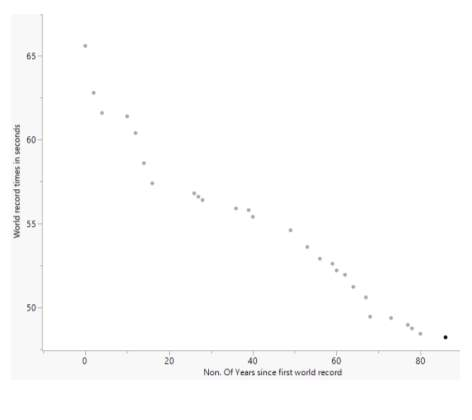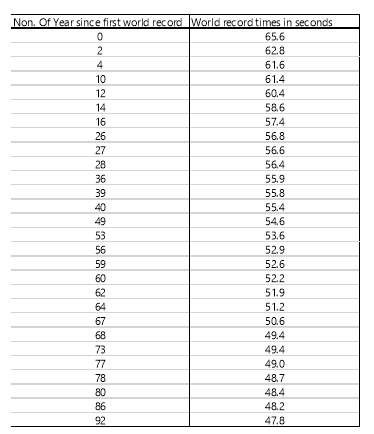Graph 1: Is a scatterplot of the men’s data set

Table 1: Is the men data set, with all the exclusion/adjustment that will be used as the basis of our models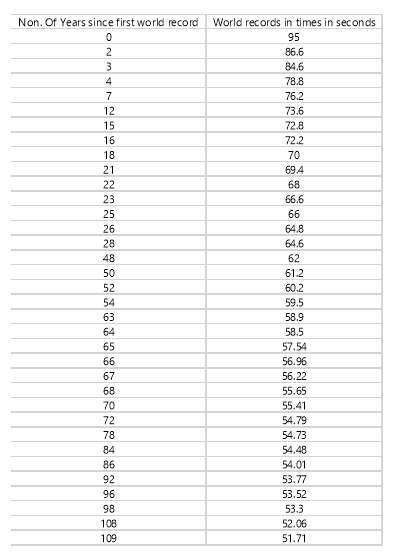Table 2: Is the women data set, with all the exclusion/adjustment that will be used as the basis of our models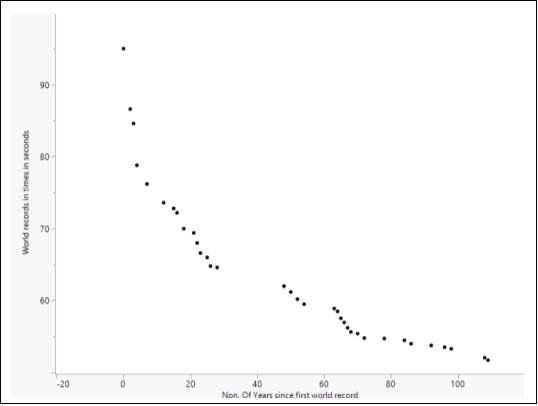Graph 2: Is a scatterplot of the women’s data set

### LINEAR FUNCTION

A linear equation or linear model represent our most simplistic predictive model for our data.

It generally written in form of:

$\mathbit{y}\mathbit{=}\mathbit{mx}\mathbit{+}\mathbit{b}$

Where m= to the gradient, rate of change or in this case the rate of the world records as the number of years passes.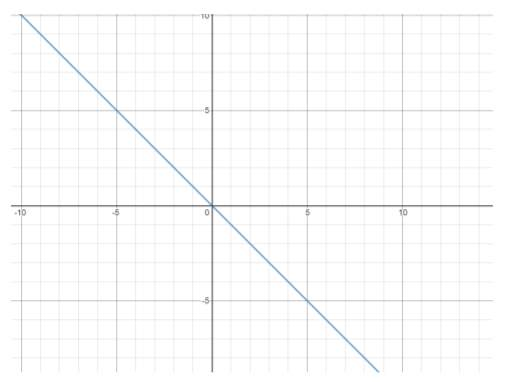B=to the y intercept or in this case the initial first world record

Graph 3: Is a basic linear equation which equal to y=-x where m=-1 and b=0

The fact that the linear equation is such a simplistic model also means it potentially has weakness. One of these is that the gradient or m represent a constant rate of change. This might affect the accuracy of the model cause we can notice in our data setsthat the world record doesn’t change at a constant rate. When analysing the domain, and range we see that the range for our linear model would be b (whatever they y-intercept is), to negative infinity. This means the limits and result may be considered unreasonable cause no one can swim negative seconds.

A quadratic function is a function whose rule may be written in the form ${\mathbit{f}}\left({\mathbit{x}}\right){\mathbit{=}}{\mathbit{a}}{{\mathbit{x}}}^{{\mathbit{2}}}{\mathbit{+}}{\mathbit{bx}}{\mathbit{+}}{\mathbit{c}}$

where a, b, and c are real numbers and a is not zero.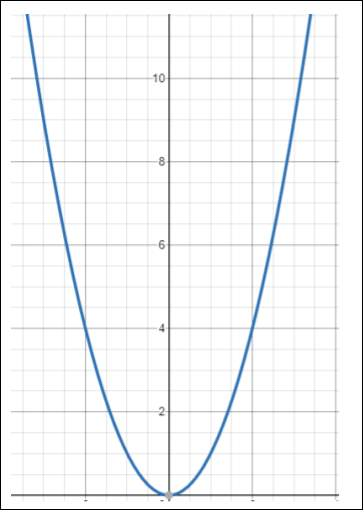The quadratic function has the possible of fitting our data more accurately compared to a linear equation. The reason for this is unlike the linear equation the quadratic has an ever-changing gradient/rate of change unlike the linear which has a constant gradient. This means that the quadratic model has the potential to better replicate the variation in the rate of change of or world record times that can be seen in our data sets. The quadratic would also give us a maximum (fastest possible world record times) or what we could call a limit which would ascertained by looking at the y value of the turning point. However, a problem would arise if the quadratic function was used as our model. The fact is after the turning point the function than ascends meaning the record would get slower which would be an unreasonable prediction.

Graph 4: Is a basic quadratic function in the form f(x)=x2 where a =1 b=0 and c=0

### EXPONENTIAL FUNCTION

In mathematics, an exponential function is a function of the form $\mathbit{f}\left(\mathbit{x}\right)\mathbit{=}\mathbit{a}{\mathbit{b}}^{\mathbit{x}}$

, where b is a positive real number, and in which the argument x occurs as an exponent.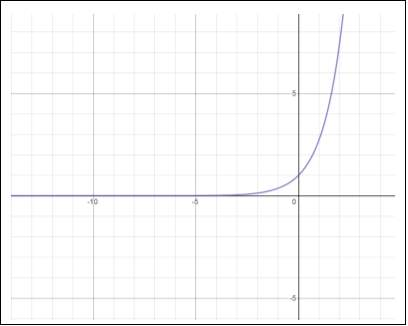An exponential function could fit my data because it has constantly decreasing rate change. This means it can replicate our data due to that fact as time (x value) passes the rate at which the world records are broken would decrease at slower rate. An exponential function also contains a horizontal asymptote, which means as x approach infinity there’s a limit to the corresponding the y values. This means that we can potentially ascertain a reasonable limit for the world record time of 100m freestyle. The exponential function contains some weakness however. If we look at our data sets we can see that the data continuously goes down than flattens out than decreases again. Unfortunately, the exponential function wouldn’t be able to effectively replicate this type of variation, which might decrease the accuracy of the model.

Graph 5: Is a basic exponential function, ex where a=0 and b = e (Euler number)

### LOGRAMTHIC FUNCTION

In mathematics, a logarithmic function is the inverse of the exponential function in is the general form of ${\mathbit{log}}_{\mathbit{a}}\mathbit{x}$

where a > 0.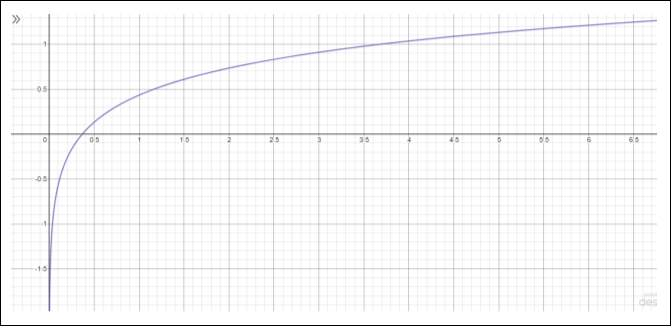As the log function is simply the inverse of the exponential function so it represents data in a similar fashion. But unlike the exponential function the log function has vertical asymptote which means it approaches a limit on the y axis. Which means it will be difficult to ascertain a limit to 100m world record time if the log function is used. It will however be difficult to use the log function as our model because when x =0 the log function would be undefined. This rectified by simply changing zero to a small decimal.

Graph 6: Is a graph of the natural logarithm ${\mathbit{log}}_{\mathbit{e}}\mathbit{x}$

### COSINE FUNCTION

A cosine function is one of the periodic trigonometric function and is usually in the general form

where A= to the amplitude, B= dilation, C=the horizontal shift and D=to the vertical shift.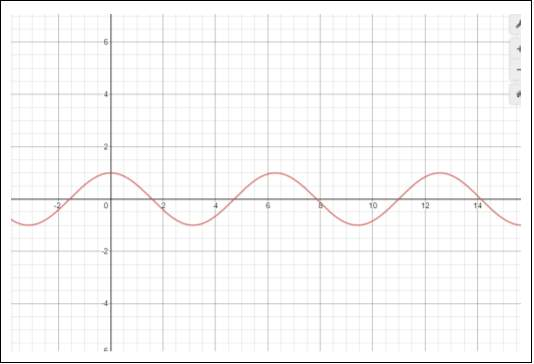The cosine function has the potential to fit the data sets due to the fact it starts at a peak and gradually decrease up until it reaches its minimum. The minimum point would represent where our limit exists (or the fastest world record). We will however have to set a domain range to insure our model doesn’t represent an unreasonable world record times.

Graph 7: Is a cos function with equation,cosx

### SIN FUNCTION

The sin function is another periodic function which is in the general form of $\mathbit{Acos}\left(\mathbit{Bx}\mathbit{+}\mathbit{C}\right)\mathbit{+}\mathbit{D}$

. A= to the amplitude, B= dilation, C=the horizontal shift and D=to the vertical shift.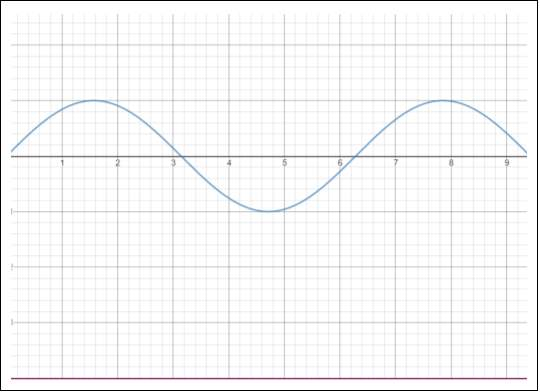The sine function is similar to cosine in the way it can represent our data set. Unlike the cosine function however the sine function starts at the point (0,0) which means we will have to manipulate it in such a way so it starts at a peak. Like the cosine function will be able to ascertain the limit to the world records by simply looking at the y value of the minimum point. We will also have to set a domain and range so the model won’t give us any results that may be considered unreasonable.

Graph 8: is a sin curve with the equation sinx

### TANGENT FUNCTION

The tangent function is another periodic function and its general form is $\mathbit{Acos}\left(\mathbit{Bx}\mathbit{+}\mathbit{C}\right)\mathbit{+}\mathbit{D}$

. A= to the amplitude, B= dilation, C=the horizontal shift and D=to the vertical shift.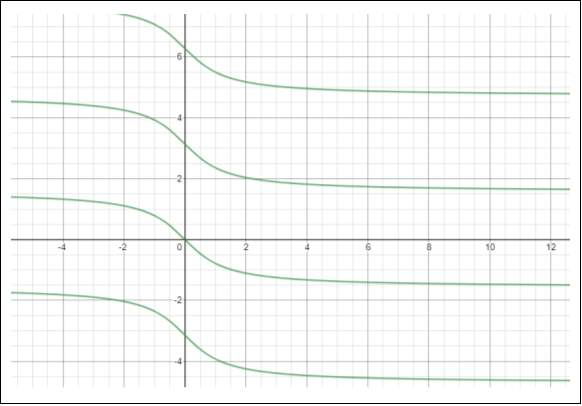The tangent function has the potential to fit my data due to the fact if its manipulated it can graph gradual decrease of y values as x values and an eventual flattening out of the data. Unlike the other periodic function, the tangent function contains horizontal asymptotes, which means we can find the limit of the world records as time (x) approaches infinity. Just like the other two periodic function it will probably required to set domain and range to the stop the repeating nature of the function.

Graph 9: is a tangent function which has been manipulated, the function is equal to $\mathbit{–}\mathbit{x}\mathbit{=}\mathbit{tany}$

### SIGMOID FUNCTION

A sigmoid function is a set of mathematical function which have a characteristic “S”-shaped curve or sigmoid curve. When discussing sigmoid function, we usually refer to the special case of the logistic function which is defined by the formula $\frac{{\mathbit{e}}^{\mathbit{x}}}{{\mathbit{e}}^{\mathbit{x}}\mathbit{+}\mathbit{1}}$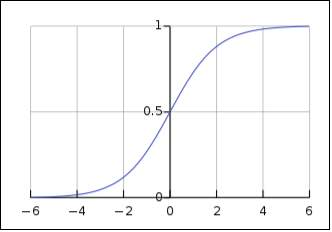Sigmoid function are bounded by 2 horizontal asymptotes which means we can obtain limit to world record times for 100m freestyle. The fact that sigmoid function initial start flat then concave (up or down depending on how its manipulated) means that it represents the initial slow rate of records broken than also represent an acceleration in world records broken (as technology improved) than finally represent the slow down once again.

Graph 10: Is a logistic curve

Sigmoid function take a variety of forms and are large set of function that can be used to represent our data and their as follow:

#### Logistic Function

A logistic function as stated before is a common sigmoid function and its general form is:

$\mathbit{f}\left(\mathbit{x}\right)\mathbit{=}\frac{\mathbit{L}}{{\mathbit{1}\mathbit{+}\mathbit{e}}^{\mathbit{–}\mathbit{k}\left(\mathbit{x}\mathbit{–}{\mathbit{x}}_{\mathbit{0}}\right)}}$

Where

• ${\mathbit{x}}_{\mathbit{0}}\mathbit{=}\mathbit{the x value of the sigmoi}{\mathbit{d}}^{\mathbit{‘}}\mathbit{smidpoint}$
• $\mathbit{k}\mathbit{=}\mathbit{the logistic growth rate or steepness of the curve}\mathbit{.}$

#### Generalised logistic function

The generalised logistic function, also known as Richard’s curve, is an extension of the logistic function, allowing for more flexible sigmoid curve. It general form is:

Unlike the logistic function it also has five parameters which are as follow:

• $\mathbit{A}\mathbit{=}\mathbit{lower asymptote}$
• $\mathbit{B}\mathbit{=}\mathbit{the growth rate}$
• $\mathbit{v}\mathbit{>}\mathbit{0}\mathbit{:}\mathbit{affects near which asymptote maximum growth occurs}$
• $\mathbit{Q}\mathbit{:}\mathbit{is related to the intial value Y}\left(\mathbit{0}\right)$

#### Gompertz Function

Gompertz function or Gompertz curve is a special sigmoid function because the right-hand or future value asymptote of the function is approached much more gradually by the curve than the left-hand or lower valued asymptote. In comparison to the simple logistic function which approaches both asymptotes symmetrically. Its general formula is:

$\mathbit{f}\left(\mathbit{x}\right)\mathbit{=}\mathbit{a}{\mathbit{e}}^{{\mathbit{–}\mathbit{be}}^{\mathbit{–}\mathbit{cx}}}$

Where,

• $\mathbit{a}\mathbit{=}\mathbit{to the asymptote}$
• $\mathbit{c}\mathbit{=}\mathbit{to the growth rate}$
• $\mathbit{e}\mathbit{=}\mathbit{to euler number}$

### HYBRID FUNCTION

In mathematics, a hybrid function (also called a piecewise) is a function defined by multiple sub function each sub-function applying to a certain interval of the main function’s domain, a sub-domain. A hybrid function would be a good model because it allows us to use a variety of other function in different domain. Which means we can use some functions which fit a part of our data set well and use another function which fit other parts of our data well. It will simply allow for greater flexibility.

## CONSTRUCTION OF MODELS-MEN

In this section of the report the constructions of the models (men) will be discussed and we will analyse the strength and limitation of the different models. We will also identify any assumption that need to be made for our models to be valid.

### ASSUMPTIONS

It is assumed that no new regulations were introduced. This due to the fact any future regulations that would be introduce could possibly impact the world record times. It is also assumed that all electronic timing machines still only record times in hundredths of seconds. Our last assumption is that; we assume all external variables have little impact on the world record times past present and future.

### LINEAR MODEL-MEN (BY HAND)

Our first model is a linear regression model which has been constructed by hand and it’s the general form is y=mx +b. In this case our m is equal to:

$\mathbit{m}\mathbit{=}\frac{\left(\mathbit{N}{\mathbit{xy}}_{\mathbit{sum}}\right)\mathbit{–}\mathbit{\left(}{\mathbit{x}}_{\mathbit{sum}}{\mathbit{x}}_{\mathbit{sum}}\mathbit{\right)}}{\left(\mathbit{N}{{\mathbit{x}}^{\mathbit{2}}}_{\mathbit{sum}}\right)\mathbit{–}\mathbit{\left(}{\mathbit{x}}_{\mathbit{sum}}{\mathbit{x}}_{\mathbit{sum}}\mathbit{\right)}}$

Where:

• ${\mathbit{x}}_{\mathbit{sum}}\mathbit{=}\mathbit{The sum of all the values in the x column}\mathbit{.}$
• ${\mathbit{y}}_{\mathbit{sum}}\mathbit{=}\mathbit{The sum of all the values in the y column}\mathbit{.}$
• ${{\mathbit{y}}^{\mathbit{2}}}_{\mathbit{sum}}\mathbit{=}\mathbit{The total of each value in the y column squared and then added together}\mathbit{.}$

And b is equal to:

$\mathbit{b}\mathbit{=}\frac{\left({{\mathbit{x}}^{\mathbit{2}}}_{\mathbit{sum}}{\mathbit{y}}_{\mathbit{sum}}\right)\mathbit{–}\mathbit{\left(}{\mathbit{x}}_{\mathbit{sum}}{\mathbit{xy}}_{\mathbit{sum}}\mathbit{\right)}}{\left(\mathbit{N}{{\mathbit{x}}^{\mathbit{2}}}_{\mathbit{sum}}\right)\mathbit{–}\mathbit{\left(}{\mathbit{x}}_{\mathbit{sum}}{\mathbit{x}}_{\mathbit{sum}}\mathbit{\right)}}$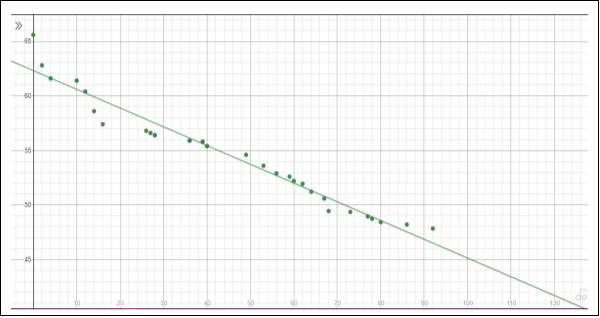After plugging the values from Table 1, we found that m=-0.1718932 and b=62.321052

Graph 11: Is our linear regression line with the equation, $\mathbit{y}\mathbit{=}\mathbit{–}\mathbit{0}\mathbit{.}\mathbit{1718932}\mathbit{x}\mathbit{+}\mathbit{62}\mathbit{.}\mathbit{321052}$

. It has a R2 value of 0.9583791 and an SSE of 27.039971

#### Strength and Limitations

Graph 11 shows that a linear fit of our men’s world record times through the first 92 year isn’t bad. This can be further corroborated by table 3 which compares the table of values of the actual data and the predicted data. Graph 12 also showcase the actual world record and the world record using the linear, it highlights the fact that they is really only one significant and large variation between the actual world record and the world record obtained by the linear equation. The linear function is however limited when attempting to ascertain limit. This is cause the graph indicates that the world record times would continue to decrease, even to the point it reaches 0. This means that an athletic could swim 100m freestyle faster than the speed of light, which is unreasonable.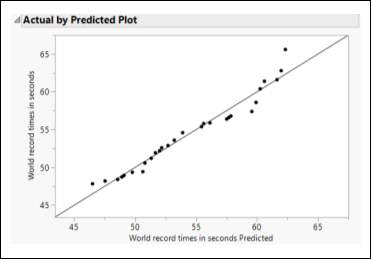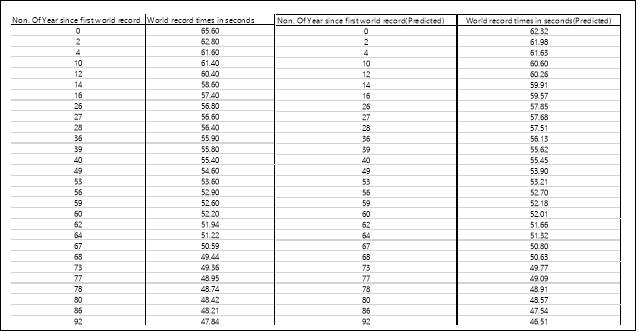Table 3: Is comparison of the actual data (left) and the data set using our linear model(right)

Graph 12: Is plot of the actual y response of the data (y axis) by the response of the predicted data (using the model, x axis). A good fit is indicated by more points being near the diagonal.

As stated in the prediction section of the report quadratic function are usually in the form of $\mathbf{a}{\mathbf{x}}^{\mathbf{2}}\mathbf{+}\mathbf{bx}\mathbf{+}\mathbf{c}$

.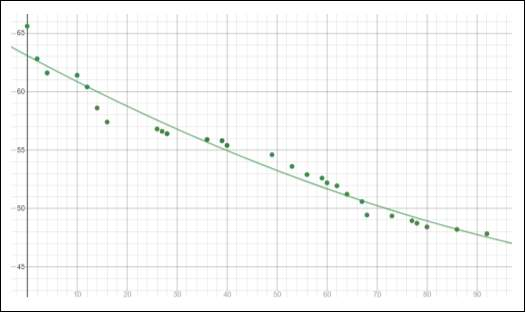Graph 13: Is our quadratic model and its equation is, $\mathbit{y}\mathbit{=}\mathbit{.}\mathbit{000659572}{\mathbit{x}}^{\mathbit{2}}\mathbit{+}\mathbit{–}\mathbit{0}\mathbit{.}\mathbit{229505}\mathbit{x}\mathbit{+}\mathbit{63}\mathbit{.}\mathbit{0802}$

. It has an R2 value of 0.9663 and a SSE of 21.8819

#### Strength and Limitations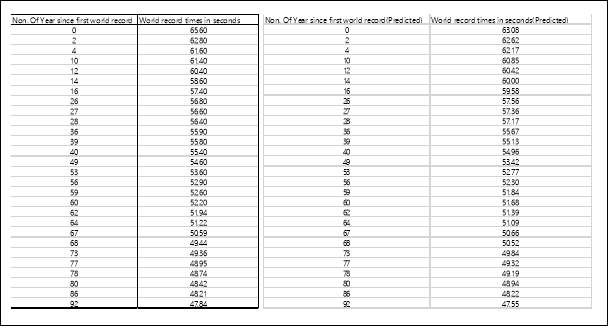Similar to the linear model the quadratic model also showcase a good fit to the data set (indicated by graph and table below) with an r squared value higher than 0.95. It also has a lower bound limit, which is a reasonable 43.16 secs that would hypothetically be hit in the year 2081. The problem is that the function begins to ascend after the plateau which means the result after the year 2081 would be unrealistic.

Table 4: Is comparison of the actual data (left) and the data set using our quadratic model(right)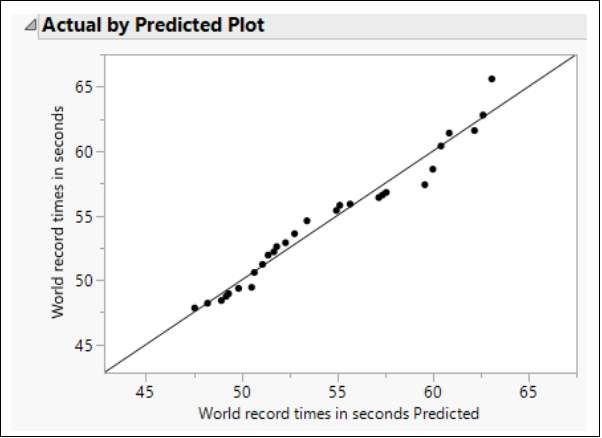Graph 14: Is plot of the actual y response of the data (y axis) by the response of the predicted data (using the model, x axis). A good fit is indicated by more points being near the diagonal.

### CUBIC MODEL-MEN (USING TECHNOLOGY)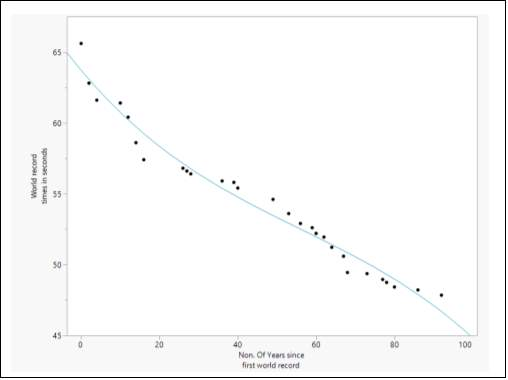Graph 15: Is a cubic function with the equation, $\mathbit{y}\mathbit{=}\mathbit{–}\mathbit{0}\mathbit{.}\mathbit{0000205014}{\mathbit{x}}^{\mathbit{3}}\mathbit{+}\mathbit{0}\mathbit{.}\mathbit{00345569}{\mathbit{x}}^{\mathbit{2}}\mathbit{+}\mathbit{–}\mathbit{0}\mathbit{.}\mathbit{329383}\mathbit{x}\mathbit{+}\mathbit{63}\mathbit{.}\mathbit{7108}$

. Its R2 value is 0.97124 and its SSE is 18.682167

#### Strength and Limitations

The cubic model provides a better representation of the data set compared to quadratic as it has a higher r squared value and a lower SSE. This also showcased by the graph ad table below. The cubic is however limited due to the fact it doesn’t provide us with any lower bound limit and the it also suggests the record would infinity decrease which is considered unreasonable.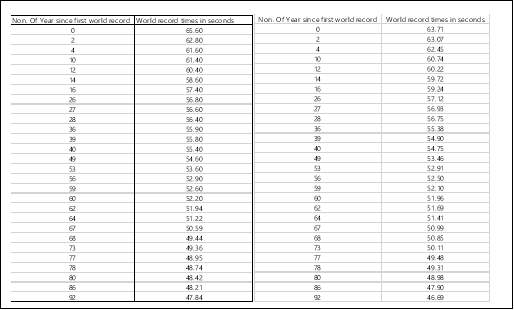Table 5: Is comparison of the actual data (left) and the data set using our cubic model(right)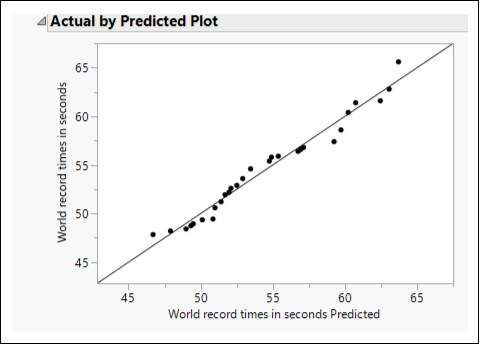Graph 16: Is plot of the actual y response of the data (y axis) by the response of the predicted data (using the model, x axis). A good fit is indicated by more points being near the diagonal.

###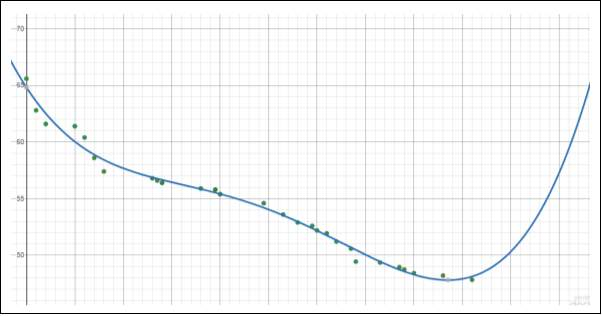QUARTIC MODEL-MEN (USING TECHNOLOGY) Graph 17: Is a quartic function (polynomial to fourth degree) with the equation, x+64.8256.It has a R2 value of 0.9878819 and a SSE of 7.8727719

#### Strengths and Limitations

The quartic model like the other model fits the data well. But its seems to almost fit the middle section of the data set perfectly. This can be seen by comparing and looking at the table below. The quartic function also has a lower bound limit at 47.81. That would have to be considered unreasonable cause it has already been surpass so it isn’t a limit. Similar to the quadratic function the quartic also begins to ascend after passing its lower bound which is seen unrealistic cause it would imply that the world record times got slower as time passed.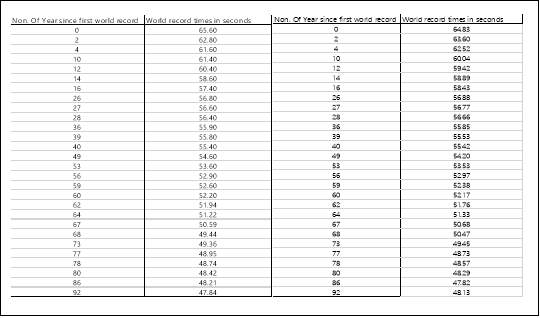Table 6: Is comparison of the actual data (left) and the data set using our quartic model(right). We can see that for the predicted data when x= 86 y=47.82 and when x=92 y=48.13 which implies over that period the record got slower which is unrealistic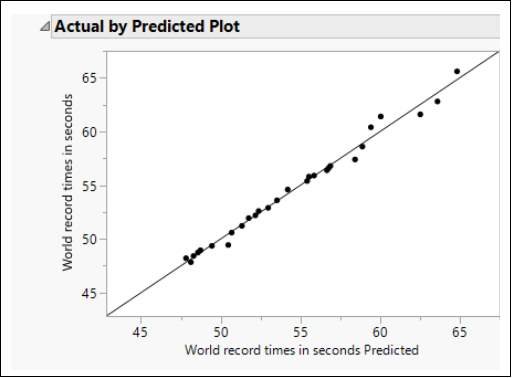Graph 18:: Is plot of the actual y response of the data (y axis) by the response of the predicted data (using the model, x axis). A good fit is indicated by more points being near the diagonal.

###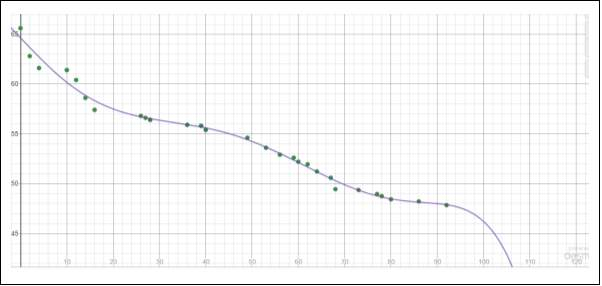POLYNOMINAL SIXITH DEGREE-MEN (USING TECHNOLOGY) Graph 19: Is a polynomial to sixth degree.  Its equation is below with the corresponding parameters.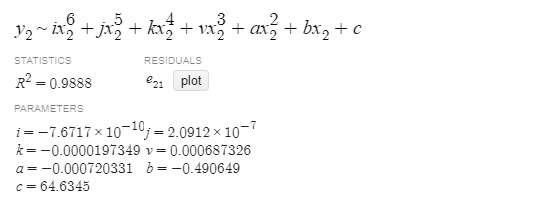#### Strength and Limitations

The polynomial to sixth degree seems to represent the middle section of my data set well even better than the quartic function. This can be corroborated by the table below comparing the actual data and the data using the model. The model however has a range of -infinity which is unreasonable and unrealistic.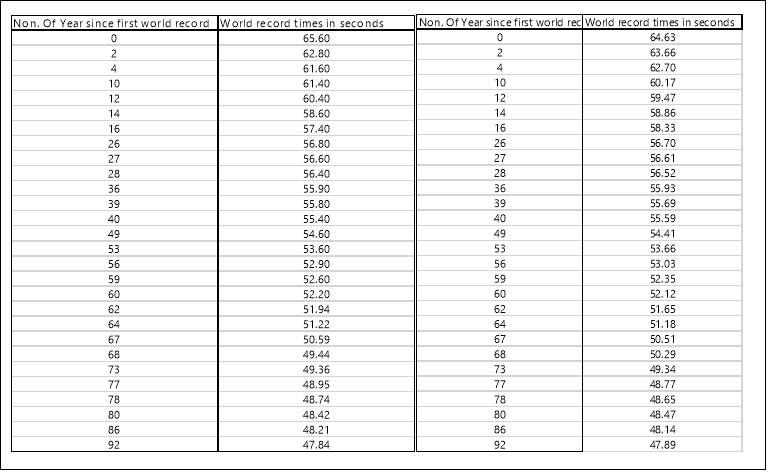Table 7:  Is comparison of the actual data (left) and the data set using our sixth-degree polynomial model(right)

### EXPOTENTIAL MODEL-MEN (USING TECHNOLOGY)

####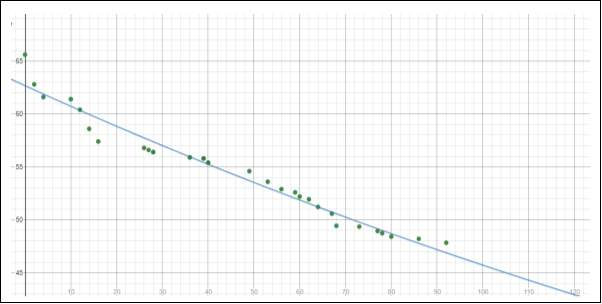Strength and Limitations Graph 20: Is an exponential model with the function, $\mathbit{y}\mathbit{=}\mathbit{62}\mathbit{.}\mathbit{6733}\mathbit{×}{\mathbit{0}\mathbit{.}\mathbit{996851}}^{\mathbit{x}}$ . It has a R2 value of 0.9636797 and a SSE of 23.596326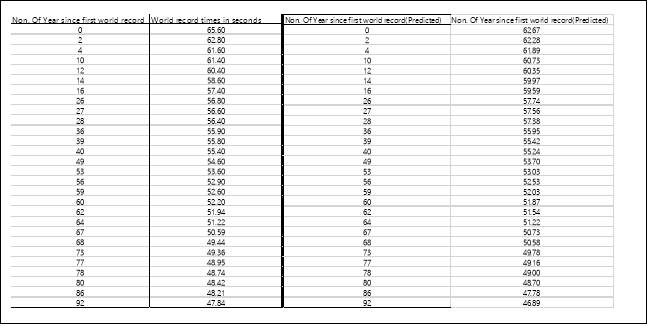The exponential decay of our model provides us with a good overall fit of our data.  It doesn’t represent any specific part of our data extremely well however. The exponential does however provide us with a limit, which is 0.996851 as x approaches infinity. Obviously, this can’t be considered as a reasonable limit to the world record times because it would imply that someone would swim 100m freestyle under 1sec.

Table 8: Is comparison of the actual data (left) and the data set using our exponential model(right)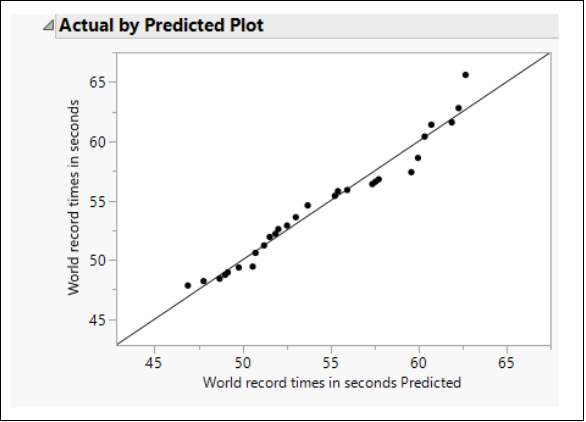Graph 21: Is plot of the actual y response of the data (y axis) by the response of the predicted data (using the model, x axis). A good fit is indicated by more points being near the diagonal.

### GOMPERTZ MODEL-MEN (USING TECHNOLOGY)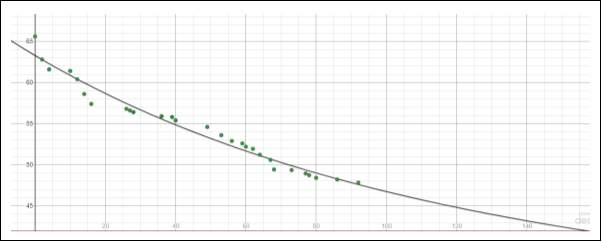As stated before the general equation for gompertz curve is: $\mathbit{f}\left(\mathbit{x}\right)\mathbit{=}\mathbit{a}{\mathbit{e}}^{{\mathbit{–}\mathbit{be}}^{\mathbit{–}\mathbit{cx}}}$

Graph 22: Is our gompertz model and has the equation,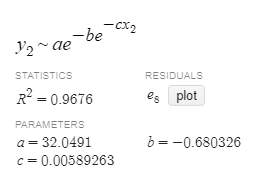#### Strength and Limitations

The gompertz model like all the other models provide us with a good representation of our data set. It doesn’t represent any part of our data set perfectly however. The gompertz also provides with a limit of 32.0491 which can be considered reasonable as its only 12 second from the current world record. When considering advancement in technology it seems completely plausible.

### GENERALISED LOGISTIC MODEL-MEN (USING TECHNOLOGY)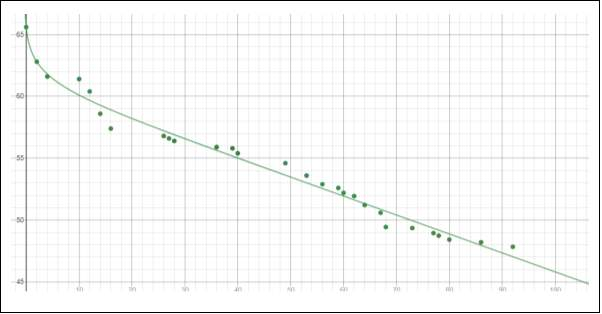As discussed the general form of generalised logistic function is:

Graph 23: Is a generalised logistic model with the equation: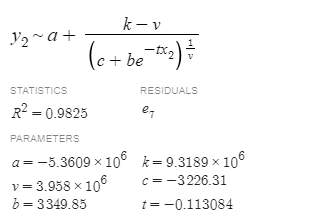$\mathbit{Y}\left(\mathbit{t}\right)\mathbit{=}\mathbit{A}\mathbit{+}\frac{\mathbit{K}\mathbit{–}\mathbit{V}}{{\mathbit{\left(}\mathbit{C}\mathbit{+}\mathbit{Q}{\mathbit{e}}^{\mathbit{–}\mathbit{Bt}}\mathbit{\right)}}^{\mathbit{1}\mathbit{/}\mathbit{v}}}$

#### Strength and Limitations

The generalised logistic model, like the other, represent the data effectively and has considerably high r squared valued. The models seem to represent the first three world record times almost perfectly from my observation. The model also has its limitation, due to the fact it indicates the world record time will infinitely decrease, which is not realistic.

### HYBRID MODEL 1-MEN (BY HAND)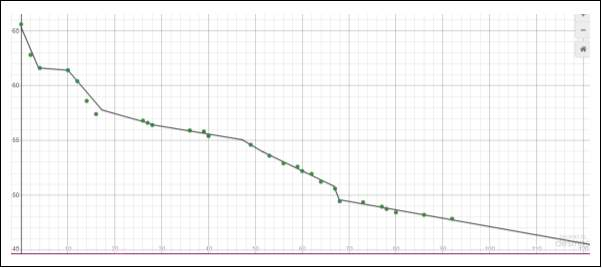This hybrid was created by hand by creating several linear functions which would fit the data set.

Graph 24: Is a hybrid model defined by domains: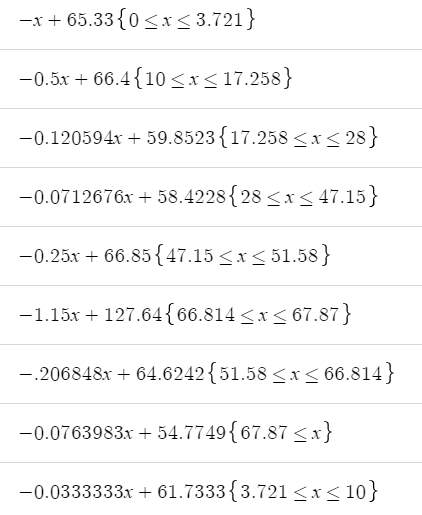#### Strength and Limitation

By using a hybrid function, I could manipulate the functions in such a way that I could create multiple linear function and simply connect them together and set domains. This has created a model that represent our data with greater accuracy. However due to the fact we used linear function, it meant that our model would indicate the limit of world record times as -infinity, which I stated multiple times is unreasonable.

### HYBRID FUNCTION 2-MEN (USING TECHNOLOGY)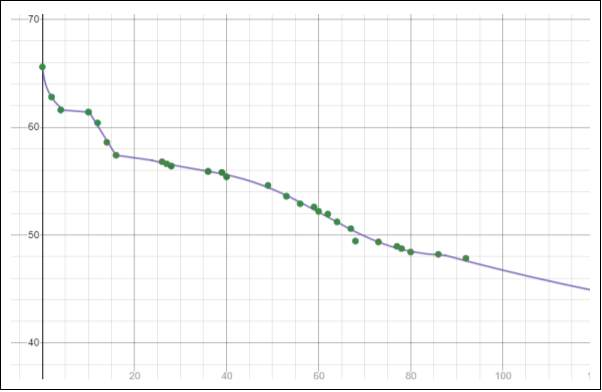My second hybrid function was thought of by looking and investigation the previous I had constructed and finding what part of the data did each model represent the best. A I also ensured that the last function I imputed had to contain a limit to infinity.

Graph 25: Is my second hybrid function which has been created by setting domains to the generalised logistic, linear function, polynomial to the sixth degree and the gompertz curve. The hybrid model is defined by the following domain:

*the domain for the polynomial is {23.809< x<87.517}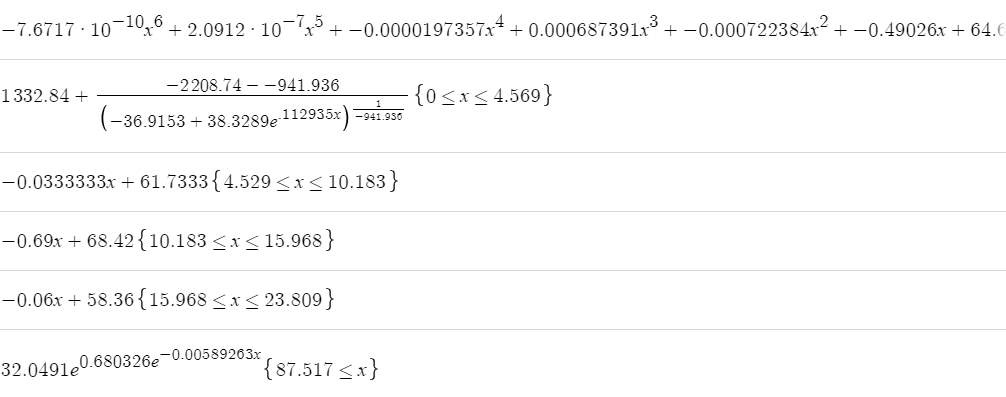#### Strength and Limitations

The hybrid model showcases and indicates all the key mission we need for our model. It represents the data well and we are also able to ascertain a limit using the model. The complexity of the model does however mean we can’t use any statistical indicator like the r squared value. We can only use observation analysis which is backed with mathematical logic.

### MATHEMATICAL ANALYSIS

 MODEL FUNCTION Y (0) = Limit R2 SSE Rank Linear 62.321 -infinity 0.9584 27.039971 10 Quadratic 63.08 infinity 0.9663 21.8819 8 Cubic 63.711 -infinity 0.9712 18.682167 7 Quartic 64.826 infinity 0.9879 7.8727719 4 Polynomial to sixth degree 64.635 -infinity 0.9888 – 5 Exponential 62.673 0.996851 0.9637 23.596326 9 Gompertz Curve 63.282 32.0491 0.9676 – 6 Generalised Logistic Curve 65.6 -infinity 0.9825 – 3 Hybrid 1 65.33 -infinity – – 2 Hybrid 2 65.57 32.0491 – – 1

Figure 1

### DISCUSSION OF BEST MODEL-MEN

Through the construction of our models of our models I found that the higher degree you go with polynomial the better the fit. The reason for this the higher the degree the more turning points meaning it can better represent fluctuations. I also realised different function fit different aspect of data better compared to other. For example, the generalised logistic model the first 3 point almost perfectly. That is how I came to conclusion of creating the hybrid function (my best model) as it combined a variety of other models and in combination fix the limitation some models had alone.

## CONSTRUCTION OF MODELS-WOMEN

In this section of the report the constructions of the models (women) will be discussed and we will analyse the strength and limitation of the different models. We will also identify any assumption that need to be made for our models to be valid.

### ASSUMPTIONS

It is assumed that no new regulations were introduced. This due to the fact any future regulations that would be introduce could possibly impact the world record times. It is also assumed that all electronic timing machines still only record times in hundredths of seconds. Our last assumption is that; we assume all external variables have little impact on the world record times past present and future.

### LINEAR MODEL -WOMEN (BY HAND)

Our first model is a linear regression model which has been constructed by hand and it’s the general form is y=mx +b. In this case our m is equal to:

$\mathbit{m}\mathbit{=}\frac{\left(\mathbit{N}{\mathbit{xy}}_{\mathbit{sum}}\right)\mathbit{–}\mathbit{\left(}{\mathbit{x}}_{\mathbit{sum}}{\mathbit{x}}_{\mathbit{sum}}\mathbit{\right)}}{\left(\mathbit{N}{{\mathbit{x}}^{\mathbit{2}}}_{\mathbit{sum}}\right)\mathbit{–}\mathbit{\left(}{\mathbit{x}}_{\mathbit{sum}}{\mathbit{x}}_{\mathbit{sum}}\mathbit{\right)}}$

Where:

• ${\mathbit{x}}_{\mathbit{sum}}\mathbit{=}\mathbit{The sum of all the values in the x column}\mathbit{.}$
• ${{\mathbit{y}}^{\mathbit{2}}}_{\mathbit{sum}}\mathbit{=}\mathbit{The total of each value in the y column squared and then added together}\mathbit{.}$

And b is equal to:

$\mathbit{b}\mathbit{=}\frac{\left({{\mathbit{x}}^{\mathbit{2}}}_{\mathbit{sum}}{\mathbit{y}}_{\mathbit{sum}}\right)\mathbit{–}\mathbit{\left(}{\mathbit{x}}_{\mathbit{sum}}{\mathbit{xy}}_{\mathbit{sum}}\mathbit{\right)}}{\left(\mathbit{N}{{\mathbit{x}}^{\mathbit{2}}}_{\mathbit{sum}}\right)\mathbit{–}\mathbit{\left(}{\mathbit{x}}_{\mathbit{sum}}{\mathbit{x}}_{\mathbit{sum}}\mathbit{\right)}}$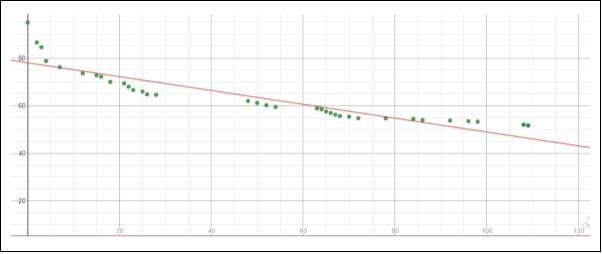after plugging the values from Table 2, we found that m=

Graph 26: Is our linear model with the equation, $\mathbit{y}\mathbit{=}\mathbit{–}\mathbit{0}\mathbit{.}\mathbit{291}\mathbit{1}\mathbit{68}\mathbit{x}\mathbit{+}\mathbit{78}\mathbit{.}\mathbit{0608}$

. It has an R2 value of 0.8203 and a SSE of 714.18697

#### Strengths and Limitations

The linear function doesn’t seem to be a good representation of the women world record times. It has considerably low r squared value (0.8203) and high SSE (714.18697). It also has a limit of -infinity which would unrealistic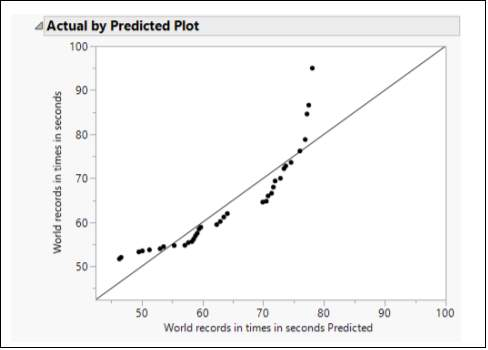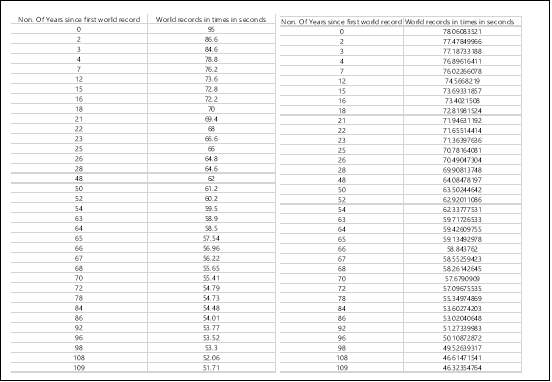Table 9: Is comparison of the actual data (left) and the data set using our linear model (right)

Graph 27:Is plot of the actual y response of the data (y axis) by the response of the predicted data (using the model, x axis). A good fit is indicated by more points being near the diagonal.

The regression equation for a quadratic equation is given by: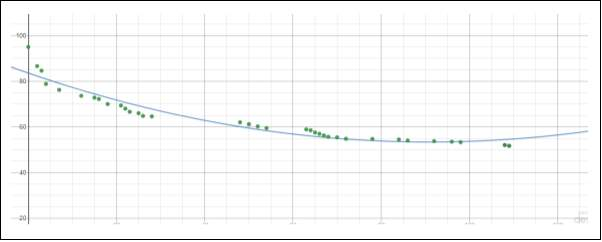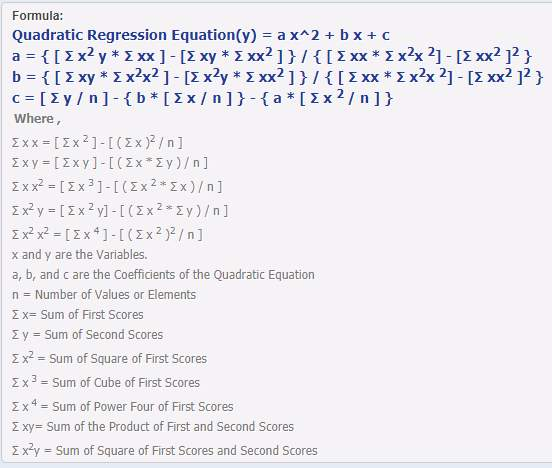Graph 28: Is a quadratic model with the equation,

. It has a R2 value of 0.9255 and a SSE of 296.09582

#### Strength and Limitations

The quadratic model is better representation of the data set compared to the linear model. I also has a lower bound limit of 53.36 seconds would could reasonable. It just means that all records that are below are simply ahead of their time. The quadratic function concave back after hitting the lower bound which means the result after the lower bound limit are unreasonable and un-logical.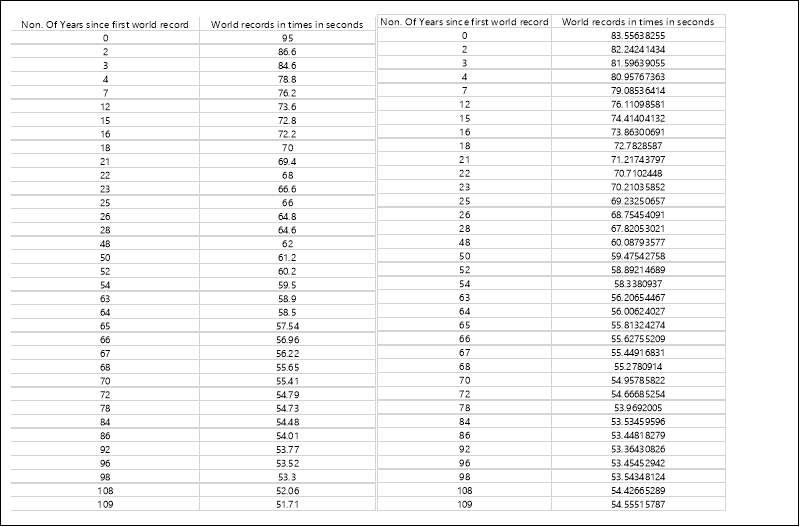Table 10: Is comparison of the actual data (left) and the data set using our quadratic model (right)

### SIXTH DEGREE POLYNOMIAL MODEL-WOMEN (USING TECHNOLOGY)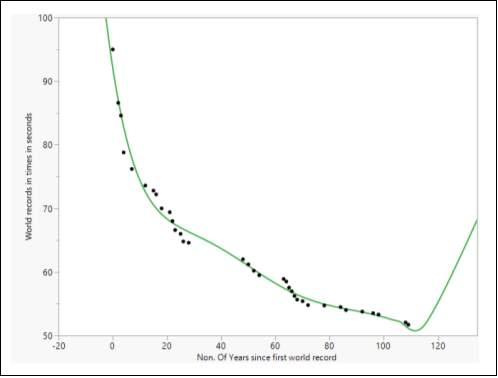Graph 29: Is our sixth-degree polynomial model with the equation,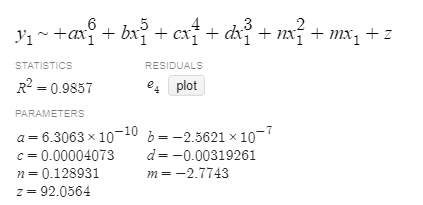#### Strength and Limitations

The sixth-degree polynomial represent our data set well, specially the start and end of the data set. It appears as if it represents those points almost perfectly. It however limited in the sense it doesn’t allow us to ascertain a limit to the world record times. The model also concave upwards which isn’t reasonable or realistic.

### EXPOTENTIAL MODEL-WOMEN (USING TECHNOLOGY)

####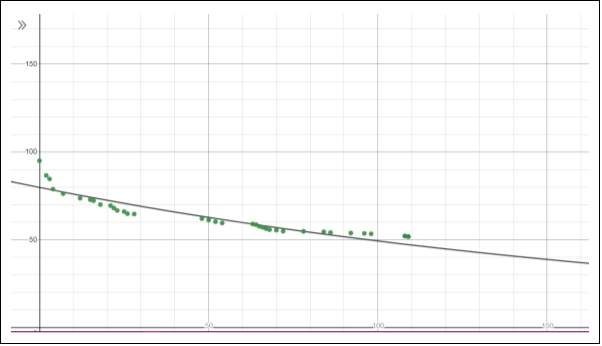Strength and Limitations Graph 30: Is an exponential model with the equation,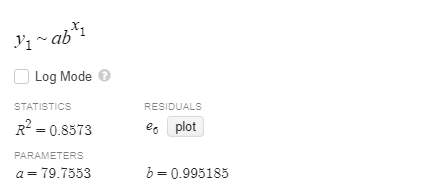The exponential decay model doesn’t really fit our data well with its low r squared value. It also provides us with an unrealistic world record limit of 0.995185.

### GOMPERTZ MODEL-WOMEN (USING TECHNOLOGY)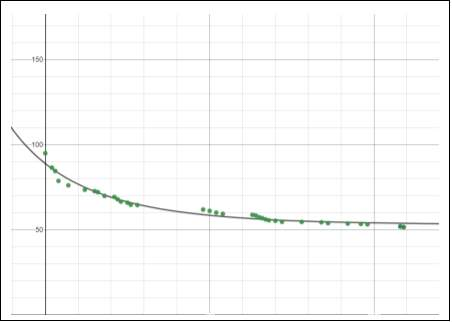Graph 31: Is a gompertz model which has the equation,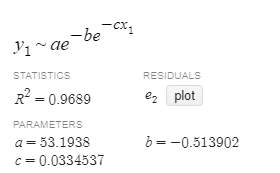#### Strength and Limitations

The gompertz model provides us we a good representation of the data set especially the middle third of the data. The model also gives us a limit to the world record of 53.1938 which can be consider semi-reasonable.

###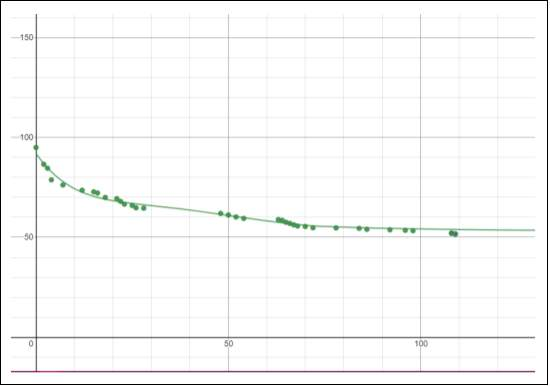HYBRIB MODEL 1-WOMEN (USING TECHNOLOGY) Graph 32: Is my hybrid model which created by using sixth degree polynomial and a gompertz function. It has the equation, the sixth-degree polynomial has a domain of {0 <x<72.326}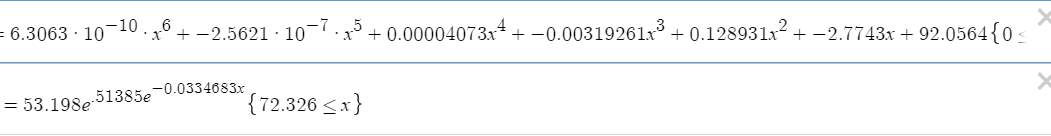#### Strength and Limitations

The hybrid function has been manipulated in such that it represents our data well using the sixth-degree polynomial while still giving a limit by using the gompertz curve. We are unable to ascertain a R squared value however so we no statistical number that tells how well the model represent the data.

### DISCUSSION OF BEST MODEL-WOMEN

I concluded the best model that represent the women’s 100m freestyle world record is hybrid 1. The reason is that I went through similar process as I did for the men. I so that the best two function that represent the data effectively was the gompertz and the sixth-degree model. The gompertz model the middle third effective while the polynomial model the start and end of the data set particularly effectively. The sixth-degree polynomial also had a limit at -infinity unlike the gompertz model which had a reasonable 53.19sec limit. So, I combined the functions and set domains to ensure the function is continuous and manipulated it to ensure that the function has a limit.

## APPLICATION OF CHOSEN MODELS

Using the models that where chosen (hybrid 2(men) and hybrid 1(women)) we can now apply them in practical environment. For instance, what would the world record time be in 2020 Tokyo Olympics. The Tokyo Olympics are 112 year from 1908 so we sub in x=112 and find the corresponding y value to find the possible world record in 2020.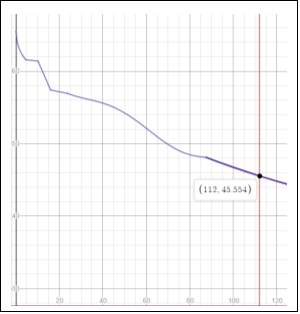Looking at graph 33 we can that men world record time in the 2020 Olympics would 45.55 seconds. This is a unreasonable result because it would a 2 second difference from the last world record, our data takes into account, of 47.84 seconds in 2000.The world record has been broken over 19 years and it’s unlikely after another year it would be broken by almost 2 seconds

Graph 33: Is the hybrid model 2(men)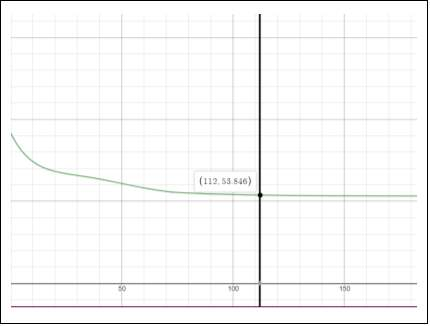Graph 34: Is the hybrid model 1(women)

Looking at graph 34 it shows that the 100m freestyle world record for women would be 53.846 seconds. This is result is unreasonable because it implies that world record goes backward in the 2020 Olympic which doesn’t make any sense.

Again, using our model on what date will women surpass the time set by men in the 2000 Olympics? We can find that world record for men by subbing x=92 and subbing that corresponding y value into the women model. Per my model the world record for men in 2000 was 47.60 second

This means that per my models women would never pass the men 2000 world record because the model for women world record times has a limit at 53.2 seconds. The result is reasonable in the sense it what my model represents. But I do find unrealistic that with future improvement in technology and training and biological makeup that women would never break the world record set by men in 2000.

Our academic experts are ready and waiting to assist with any writing project you may have. From simple essay plans, through to full dissertations, you can guarantee we have a service perfectly matched to your needs.

Last application is, on what date did men surpass the time set by women in the 2000 Olympics? We use a similar process to just before. We sub x=92 into the model for women and sub the that corresponding y value in to the model for men. According to the model for women the world record for women in the year 2000 was 54.47 seconds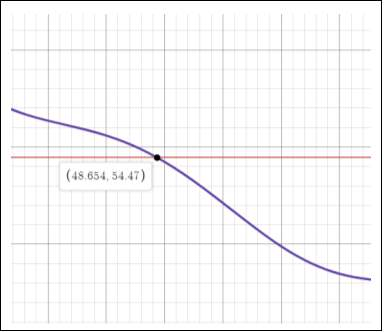This means that the men broke the world record set by women in 2000 in 1957.This is cute a reasonable result because if we compare Table 1 and 2 we see a that men broke the world record set by women in 2000 in 1961. They is only a 4-year difference.

Graph 35: Is the hybrid model 2(men)

## CONCLUSION

As stated in the introduction the question whether they would ever be a limit to world record times, isn’t the real question we should be asking. Cause if we take the extreme, it’s fair to say “Humans cannot run [or swim] at, say, the speed of light, so there must be a limit to how fast it is possible to run [or swim]” (Jay, 2016). So, the real question isn’t whether they is a limit its, can we use mathematical models to ascertain where those limits are. For example, the result for our investigation concluded that the limit for 100m freestyle time was 32.04 seconds and for women was 53.2 seconds. These results are overstated and understated respectively. The reality is that our investigation simply didn’t consider enough external variable that impact the improvement of world records such as

• “The use of more efficient running, jumping and swimming techniques, a biomechanical construct.
• Improved training programs, which are exercise physiological and functional anatomical construct.
• Enlarged population of athletes due to increased participation by more nations from which high performance athletes and swimmers are drawn. This will result in an increased sample from the human gene pool, a genetic construct.
• Improved talent identification programs designed and implemented by national sporting organisations and sports institutes that will select and develop tomorrow’s high performance athletes.
• Changes in human physiology, such as the recent ontogenetic trends of increasing height and weight in Australia.”(Heazlewood, 2006)

It also highlights an underlying limitation of mathematical models which is that they “pertains to observations made in the past, and so it can be used for policy making, and not for decision making which requires observations or situations in the present” (Ram Gopal Gupta,2016). The one consistent thing that all model showed was however continuous improvement due to all the factor above and more. Overall the investigation gave me in insight into the capabilities of human performance and our limits. It also highlighted the use of mathematical models as prediction tools not just in mathematics.

### OTHER USES OF MATHEMATICAL MODEL

As discussed in the literature review section of the report they have been many other investigations that used mathematical models to ascertain limits for world record times. For example, in finance a Gaussian function—a bell curve can predict that extreme dip and rises in the financial market would be rare and wouldn’t be prone to following on after the other on succeeding days. In medicine a similar investigation could be used to elucidate the mechanisms of a phenomenon and predict its future course. The medical sector also uses mathematical models for “planning and evaluation of preventive and control programmes, clinical trials, measurement of health, cost-benefit analysis, diagnosis of patients and in maximizing effectiveness of operations aimed at attaining specified goals within existing resources.” (Verma Bl, 2019). Predictive mathematical models can also be used in public sector by analysing and investigation population growth, which would allow policy makers to be prepared for potential increase or decrease in the city or countries population.

View all

## DMCA / Removal Request

If you are the original writer of this essay and no longer wish to have your work published on UKEssays.com then please:

Related Services

Prices from

SR571

Approximate costs for:

• 1000 words
• 7 day deliveryHumanity University

Dedicated to your worth and value as a human being!

Related Lectures